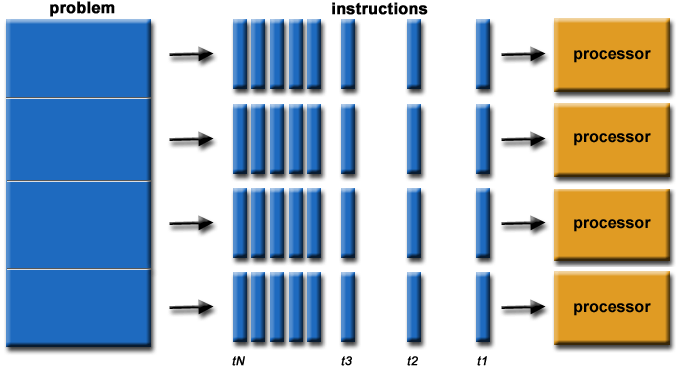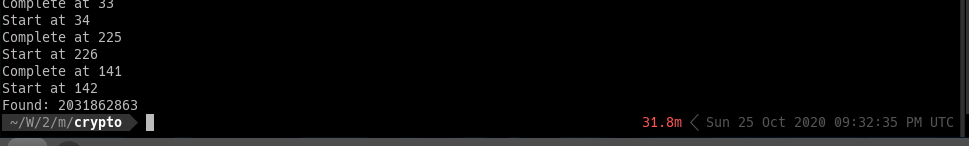# Embarrassingly Parallel Key Search With Julia

Parallel computing in Julia is so easy## Embarrassingly Parallel Key Search With Julia

Recently I play a crypto problem, the problem is quite simple, it’s general Feistel cipher construction. You can see the problem at encryption.py. This is the challenge from MetaCTF 2020.

So the attack is quite general, starts with some analysis:

• Mode of Operation is ECB

• Feistel Construction

• Offline attack, no oracle provided

• Block size is 64-bit, key size is 32-bit, say in the code.

Since the key size is 32-bit, it’s easy to do a exhaustive search 32-bit space to recover secret key.

Here was my attempts:

1. Multiple threading with Python3

2. Multiple threading with pypy3

3. Multiple threading with Julia

In 1, it took quite long to brute 25-bit.

In 2, it’s faster than 1., still slow.

In 3, it’s fastest solution.

The code for 1 and 2 can be found here: brute.py. Thanks to Zaine Wilson @ MasonCC.

The code for 3 is developed by me, stream_brute.jl. If you’re using Mobile to read my code, here is the full code.

mask32 = 0xffffffff

le = UInt32(0)
ri = UInt32(0)

le = block >> 32

for i = 1:rounds
# println("le: $i$le")
le = (le << 19) | (le >> 13)
le ⊻= ri

tmp = ri
ri = le
le = tmp

end

ciphertext = le << 32
ciphertext |=  ri

return ciphertext

end

known_pt = UInt64(0x4141414141414141)
known_ct = UInt64(0x212ced02de0ba3d5)

function break_routine(start)
println("Start at $start") pw = UInt64(0) p1 = UInt8(start) for p2 = 0:256 for p3 = 0:256 for p4 = 0:256 pw = UInt64(p1) << 24 pw |= UInt64(p2) << 16 pw |= UInt64(p3) << 8 pw |= UInt64(p4) temp = encryptblock(known_pt, pw, 16) if temp == known_ct println("Found:$pw")
exit(0)
end
end
end
end

println("Complete at \$start")
end

# Check with Python, make sure it correct
key = UInt64(0x44424344)
ciphertext = encryptblock(known_pt, key, 16)
if ciphertext != 4357724131518883252
println("WRONG!!! Check the code")
exit(1)
end

println("PASS, start to brute... .")
# asyncmap(break_routine, range(0, length=256, step=1), ntasks=12)
break_routine(i)
end

# julia stream_brute.jl

To run the code, if you are using Julia version < 1.5, then you may have to do export JULIA_NUM_THREADS=12 where 12 is number of thread in my system. Then run julia stream_brute.jl

If your Julia version is >= 1.5, then just run julia stream_brute.jl --threads 12

The code above can be improved further more, since I’m new with Julia so that’s all I know.

Isn’t it impressive if we can do parallel tasks this easy?

Threads.@threads for i = 0:255
break_routine(i)
end

As conversation with Zaine, his Python version run in 6+ hours.

My script run in 0.5 hour. So that is 12x speed up. All scripts are multithreading.## Conclusion

Embarrassing Parallel in Julia is so easy, the syntax is pretty like Python.

One thing I need to keep in mind though, array start at 1.

## Challenge

If you want to test the performance of Julia in your machine, try to decrypt this my message.

97b0f603bc78c5b215f8404f9f3532bfab75df10bb966fc35b7d8a217e07f5c17f32b158b1b5eb61f1bbe86a47daffa0811b63e0baf5aaab83becd22ef0b24e15011e9901cb4c408b39aa36efc81a63f88fde01463ec013c4911add72947a5da4ca667125061057e4f8e82c018022fbf2658696b32f9c2f1a6e12b51379bdb544f664521053b92ced32674d0d537c4d57913252a05f7637baba004b766a9f7213cf39590b28abb6e4aeb86bbae1653e7381447acd9fdaaea7dff1cd2bb25

My padding char is E

The code is similar to encryption.py

Have fun.##### Cothan
###### Cryptography Engineering

My research interests include implementation of Post-Quantum Cryptography using High Level Synthesis in FPGA and NEON instruction in ARM platform, beside, sometimes I play CTFs, Crypto and Reverse Engineering are my favorite categories.